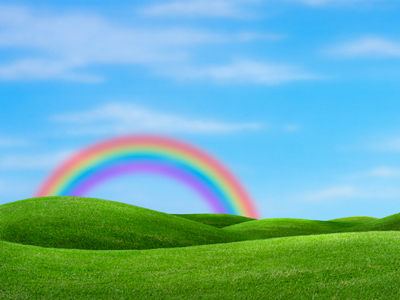Rainbows have 7 colours - red, orange, yellow, green, blue, indigo and violet.

# 7 Times Table

In KS1 Maths and Numeracy children will be exposed to the times tables as early as possible in Year 1. The 1, 2, 5 and 10 times tables are taught first before the 3, 4, 6, 7, 8 and 9 times tables follow. By the end of Year 2 children are often fluent in their times tables up to 10.

This quiz will test children's familiarity with their seven times table, which will help them in studying maths in Years 1 and 2.

Do you know your seven times table? Try this quiz and see how you get on.

1.
9 x 7 =
70
63
56
60
2.
8 x 7 =
56
55
49
57
3.
7 x 10 =
63
70
77
75
Remember, 10 times a number is that number with a 0 on its end
4.
1 x 7 =
1
0
8
7
There are 7 colours in the rainbow: red, orange, yellow, green, blue, indigo and violet
5.
0 x 7 =
1
7
0
14
Any number times by 0 = 0
6.
7 x 5 =
30
35
28
42
7 x 5 is the same as 5 x 7 so your 5 times table could help you here
7.
7 x 3 =
14
28
24
21
7 x 3 is the same as 7 + 7 + 7
8.
7 x 4 =
28
21
35
30
9.
7 x 7 =
45
42
49
56
When we multiply a number by itself we say that we have squared it, so 7 squared = 49
10.
7 x 2 =
21
14
9
7
To find 2 times a number just add it to itself, or double it
Author:  Graeme Haw